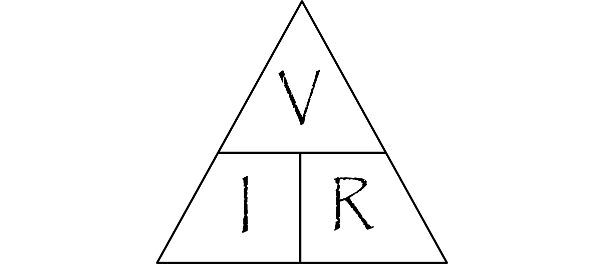# What is the electric current produced when a voltage of 9 V is applied to a circuit with a resistance of 66 Omega?

Jan 23, 2016

Current $= 136.364 \text{ mA}$

#### Explanation:

$I = \frac{V}{R}$ where $I$ is the current, $V$ is the voltage, and $R$ is the resistance.
$\textcolor{w h i t e}{\text{XX}}$Think of it this way:
$\textcolor{w h i t e}{\text{XXXX}}$If you increase the pressure (voltage), you will increase the amount of current.
$\textcolor{w h i t e}{\text{XXXX}}$If you increase the resistance, you will decrease the amount of current.Current is measured with a base unit of $A =$ ampere which is defined as the current produced by $1 V$ through a circuit with $1 \Omega$ resistance.

For the given values:
$\textcolor{w h i t e}{\text{XXX}} I = \frac{9 V}{66 \Omega}$

$\textcolor{w h i t e}{\text{XXX}} = \frac{3}{22} A = 0.136364 A$

For values in this range it is more common to specify the result in $m A$ (miliamperes) where $1000 m A = 1 A$